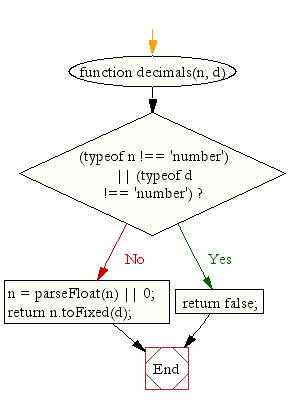# JavaScript: Format a number up to specified decimal places

## JavaScript Math: Exercise-5 with Solution

Write a JavaScript function to format a number up to specified decimal places.

Test Data :
console.log(decimals(2.100212, 2));
console.log(decimals(2.100212, 3));
console.log(decimals(2100, 2));
"2.10"
"2.100"
"2100.00"

Pictorial Presentation:Sample Solution:-

HTML Code:

``````<!DOCTYPE html>
<html>
<meta charset="utf-8">
<title>JS Bin</title>
<body>

</body>
</html>
```
```

JavaScript Code:

``````function decimals(n, d) {
if ((typeof n !== 'number') || (typeof d !== 'number'))
return false;
n = parseFloat(n) || 0;
return n.toFixed(d);
}
console.log(decimals(2.100212, 2));
console.log(decimals(2.100212, 3));
console.log(decimals(2100, 2));
```
```

Sample Output:

```2.10
2.100
2100.00
```

Flowchart:Live Demo:

See the Pen javascript-math-exercise-5 by w3resource (@w3resource) on CodePen.

Improve this sample solution and post your code through Disqus

What is the difficulty level of this exercise?

Test your Programming skills with w3resource's quiz.

﻿